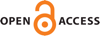Download this articleFor screen For printingRecent IssuesThe Journal About the Journal Editorial Board Editors’ Interests Subscriptions Submission Guidelines Submission Form Policies for Authors Ethics Statement ISSN: 1948-206X (e-only) ISSN: 2157-5045 (print) Author Index To Appear Other MSP JournalsTalagrand's influence inequality revisited

### Dario Cordero-Erausquin and Alexandros Eskenazis

Vol. 16 (2023), No. 2, 571–612##### Abstract

Let ${\mathsc{𝒞}}_{n}={\left\{-1,1\right\}}^{n}$ be the discrete hypercube equipped with the uniform probability measure ${\sigma }_{n}$. Talagrand’s influence inequality (1994), also known as the ${L}_{1}-{L}_{2}$ inequality, asserts that there exists $C\in \left(0,\infty \right)$ such that for every $n\in ℕ$, every function $f:{\mathsc{𝒞}}_{n}\to ℂ$ satisfies

 ${\mathrm{Var}}_{{\sigma }_{n}}\left(f\right)\le C\sum _{i=1}^{n}\frac{\parallel {\partial }_{i}f{\parallel }_{{L}_{2}\left({\sigma }_{n}\right)}^{2}}{1+\mathrm{log}\left(\parallel {\partial }_{i}f{\parallel }_{{L}_{2}\left({\sigma }_{n}\right)}∕\parallel {\partial }_{i}f{\parallel }_{{L}_{1}\left({\sigma }_{n}\right)}\right)}.$

We undertake a systematic investigation of this and related inequalities via harmonic analytic and stochastic techniques and derive applications to metric embeddings. We prove that Talagrand’s inequality extends, up to an additional doubly logarithmic factor, to Banach space-valued functions under the necessary assumption that the target space has Rademacher type 2 and that this doubly logarithmic term can be omitted if the target space admits an equivalent 2-uniformly smooth norm. These are the first vector-valued extensions of Talagrand’s influence inequality. Moreover, our proof implies vector-valued versions of a general family of ${L}_{1}-{L}_{p}$ inequalities, each refining the dimension independent ${L}_{p}$-Poincaré inequality on $\left({\mathsc{𝒞}}_{n},{\sigma }_{n}\right)$. We also obtain a joint strengthening of results of Bakry–Meyer (1982) and Naor–Schechtman (2002) on the action of negative powers of the hypercube Laplacian on functions $f:{\mathsc{𝒞}}_{n}\to E$, whose target space $\left(E,\parallel \cdot {\parallel }_{E}\right)$ has nontrivial Rademacher type via a new vector-valued version of Meyer’s multiplier theorem (1984). Inspired by Talagrand’s influence inequality, we introduce a new metric invariant called Talagrand type and estimate it for Banach spaces with prescribed Rademacher or martingale type, Gromov hyperbolic groups and simply connected Riemannian manifolds of pinched negative curvature. Finally, we prove that Talagrand type is an obstruction to the bi-Lipschitz embeddability of nonlinear quotients of the hypercube ${\mathsc{𝒞}}_{n}$ equipped with the Hamming metric, thus deriving new nonembeddability results for these finite metrics. Our proofs make use of Banach space-valued Itô calculus, Riesz transform inequalities, Littlewood–Paley–Stein theory and hypercontractivity.

##### Keywords
Hamming cube, Talagrand's inequality, Rademacher type, martingale type, Itô calculus, Riesz transforms, Littlewood–Paley–Stein theory, hypercontractivity, CAT(0) space, bi-Lipschitz embedding
##### Mathematical Subject Classification
Primary: 42C10
Secondary: 30L15, 46B07, 60G46
##### Milestones